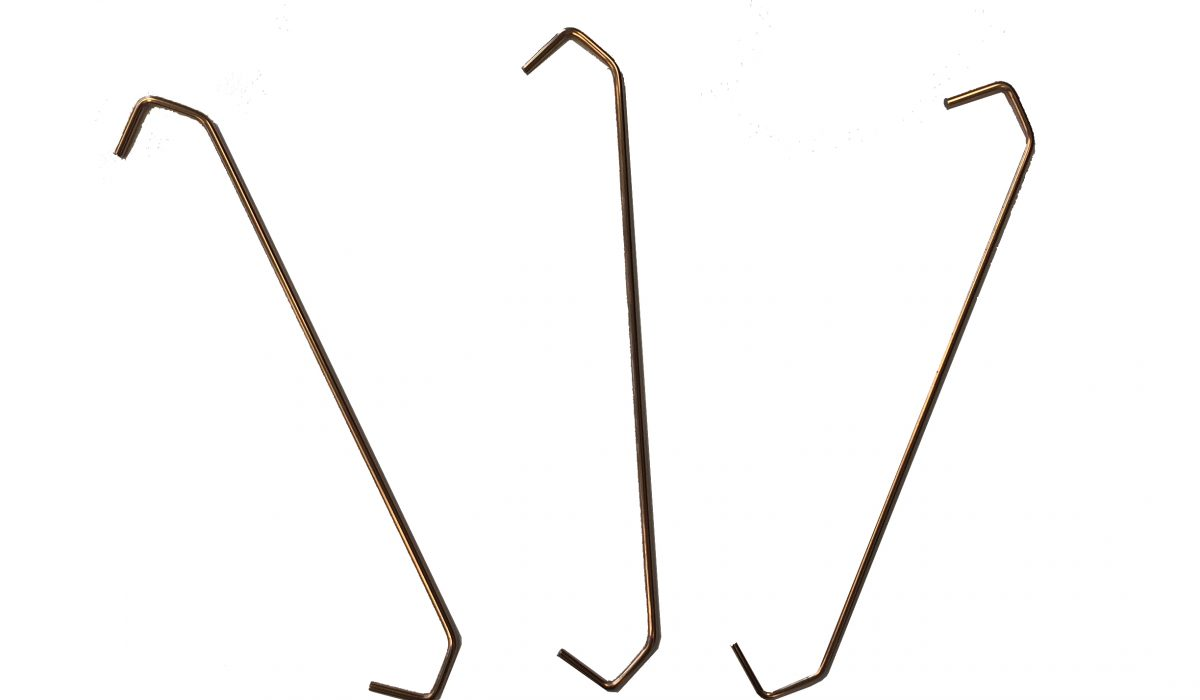27 JunTypical dynamic behaviour of standard hooks when loaded is that maximum load, the load where the wire starts to be permanently deformed (bending strength) is the same for DV and R but approximately double for DV M.
Then DV and DV M describes a “concave” load-extension line before the load falls or drops off, while R looks more like a convex figure.

Load capacity of a single hook is dependent on many things, the most important is material, wire diameter and orthogonal distance. An approximate formula true for a single hook:

Mmax ≈  σs * ø³/99,5e

Mmax = load where irreversible deformation starts (kg)

σs = bending strength (N/mm2)

e = orthogonal distance (mm)Typical dynamic behaviour of standard hooks when loaded is that maximum load, the load where the wire starts to be permanently deformed (bending strength) is the same for DV and R but approximately double for DV M.
Then DV and DV M describes a “concave” load-extension line before the load falls or drops off, while R looks more like a convex figure.